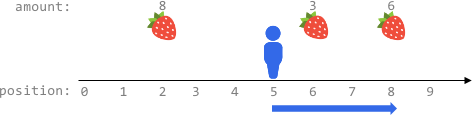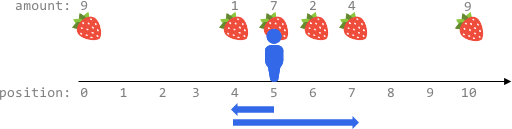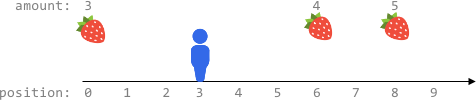##### Welcome to Subscribe On Youtube

Formatted question description: https://leetcode.ca/all/2106.html

# 2106. Maximum Fruits Harvested After at Most K Steps (Hard)

Fruits are available at some positions on an infinite x-axis. You are given a 2D integer array fruits where fruits[i] = [positioni, amounti] depicts amounti fruits at the position positioni. fruits is already sorted by positioni in ascending order, and each positioni is unique.

You are also given an integer startPos and an integer k. Initially, you are at the position startPos. From any position, you can either walk to the left or right. It takes one step to move one unit on the x-axis, and you can walk at most k steps in total. For every position you reach, you harvest all the fruits at that position, and the fruits will disappear from that position.

Return the maximum total number of fruits you can harvest.

Example 1:Input: fruits = [[2,8],[6,3],[8,6]], startPos = 5, k = 4
Output: 9
Explanation:
The optimal way is to:
- Move right to position 6 and harvest 3 fruits
- Move right to position 8 and harvest 6 fruits
You moved 3 steps and harvested 3 + 6 = 9 fruits in total.


Example 2:Input: fruits = [[0,9],[4,1],[5,7],[6,2],[7,4],[10,9]], startPos = 5, k = 4
Output: 14
Explanation:
You can move at most k = 4 steps, so you cannot reach position 0 nor 10.
The optimal way is to:
- Harvest the 7 fruits at the starting position 5
- Move left to position 4 and harvest 1 fruit
- Move right to position 6 and harvest 2 fruits
- Move right to position 7 and harvest 4 fruits
You moved 1 + 3 = 4 steps and harvested 7 + 1 + 2 + 4 = 14 fruits in total.


Example 3:Input: fruits = [[0,3],[6,4],[8,5]], startPos = 3, k = 2
Output: 0
Explanation:
You can move at most k = 2 steps and cannot reach any position with fruits.


Constraints:

• 1 <= fruits.length <= 105
• fruits[i].length == 2
• 0 <= startPos, positioni <= 2 * 105
• positioni-1 < positioni for any i > 0 (0-indexed)
• 1 <= amounti <= 104
• 0 <= k <= 2 * 105

Similar Questions:

## Solution 1. Sliding Window

// OJ: https://leetcode.com/problems/maximum-fruits-harvested-after-at-most-k-steps/
// Time: O(N)
// Space: O(1)
class Solution {
public:
int maxTotalFruits(vector<vector<int>>& A, int start, int k) {
int N = A.size(), i = 0, sum = 0;
for (; i < N && A[i] <= start; ++i);
int j = i;
while (j < N && A[j] - start <= k) sum += A[j++];
--j;
int ans = sum;
while (i > 0) {
--i;
sum += A[i];
while (j >= i && A[j] - start + A[j] - A[i] > k) {
sum -= A[j--];
}
if (j < i) continue;
if (A[j] < start) break;
ans = max(ans, sum);
}
i = N - 1;
sum = 0;
for (; i >= 0 && A[i] >= start; --i);
j = i;
while (j >= 0 && start - A[j] <= k) sum += A[j--];
++j;
ans = max(ans, sum);
while (i < N - 1) {
++i;
sum += A[i];
while (j <= i && start - A[j] + A[i] - A[j] > k) {
sum -= A[j++];
}
if (j > i) continue;
if (A[j] > start) break;
ans = max(ans, sum);
}
return ans;
}
};


## Solution 2. Sliding Window

Note: when comparing 2 vectors, the result is determined in the following order:

1. The first place where the values differ between the two arrays.
2. If we’ve exhausted one array and still can’t have a difference, the array with longer length is greater.

In the following code, we use {start - k} in the binary search. It is always smaller than a position with the same x value {start - k, amount}. So using upper_bound or lower_bound makes no difference.

(start - A[i]) + (A[j] - A[i]) is the steps needed to go left first then right.

(A[j] - A[i]) + (A[j] - start) is the steps needed to go right first then left.

// OJ: https://leetcode.com/problems/maximum-fruits-harvested-after-at-most-k-steps/
// Time: O(N)
// Space: O(1)
// Ref: https://leetcode.com/problems/maximum-fruits-harvested-after-at-most-k-steps/discuss/1624215/(Strange)-Sliding-Window
class Solution {
public:
int maxTotalFruits(vector<vector<int>>& A, int start, int k) {
int i = upper_bound(begin(A), end(A), vector<int>{start - k}) - begin(A), sum = 0, ans = 0, N = A.size();
for (int j = i; j < N && A[j] <= start + k; ++j) {
sum += A[j];
while (min(start - 2 * A[i] + A[j], 2 * A[j] - A[i] - start) > k) sum -= A[i++];
ans = max(ans, sum);
}
return ans;
}
};